## How to remove non numeric characters from a cell

This post explains that how to remove non-numeric characters (numbers) from a text string in one cell in excel 2016/2019/365. How to remove non numeric characters from a cell containing text string with an excel formula. And how to remove alphanumeric characters from a cell with a user defined function in excel VBA.

## 1. Remove non numeric characters with an Excel Formula

If you want to remove non numeric characters from a text cell in excel, you can use the array formula:

``{=TEXTJOIN("",TRUE,IFERROR(MID(B1,ROW(INDIRECT("1:"&LEN(B1))),1)+0,""))}``

Let’s see how the above formula works:

=ROW(INDIRECT(“1:”&LEN(B1))

The ROW function returns the below array list:

``{1,2,3,4,5,6,7,8,9}``

=MID(A1,ROW(INDIRECT(“1:”&LEN(A1))),1)

The MID formula will return the below array:

``{"e","x","c","e","l","2","0","1","6"}``

=IFERROR(MID(B1,ROW(INDIRECT(“1:”&LEN(B1))),1)+0,””)

The array returned by the above MID function add zero for each value in array. If the value is a numeric text, it will be converted to text format. If not, returns empty string. So the IFERROR function returns the below array:

``{2,0,1,6}``

Last, the TEXTJOIN function join the values in above array returned by the IFERROR function.

## 2. Remove non numeric characters using VBA Code

You can create a new function to remove numeric characters from a cell that contain text string in Excel VBA. Just refer to the below steps:

Step1: open visual Basic Editor, then insert a module and name as : RemoveNonNum.

Step2: click “Insert“->”Module“, then paste the following VBA code into the window:

Step3: paste the below VBA code into the code window. Then clicking “Save” button.

```Sub RemoveNonNum()
Set myRange = Application.Selection
Set myRange = Application.InputBox("select one Range that you want to remove non numeric characters", "RemoveNonNum", myRange.Address, Type:=8)
For Each myCell In myRange
LastString = ""
For i = 1 To Len(myCell.Value)
mT = Mid(myCell.Value, i, 1)
If mT Like "[0-9]" Then
tString = mT
Else
tString = ""
End If
LastString = LastString & tString
Next i
myCell.Value = LastString
Next
End Sub```

Step4: back to the current worksheet, then run the above excel macro. Click Run button.

Step5: select one Range that you want to remove non numeric characters. click Ok button.

Step6: Let’s see the last result:

## 3. Video: Remove non numeric characters in Excel

This video will demonstrate how to remove non-numeric characters in Excel using a formula or VBA code.

## 4. Related Formulas

• Remove Numeric Characters from a Cell
If you want to remove numeric characters from alphanumeric string, you can use the following complex array formula using a combination of the TEXTJOIN function, the MID function, the Row function, and the INDIRECT function..…
• Combine Text from Two or More Cells into One Cell
If you want to combine text from multiple cells into one cell and you can use the Ampersand (&) symbol.If you are using the excel 2016, then you can use a new function TEXTJOIN function to combine text from multiple cells…

## 5. Related Functions

• Excel TEXTJOIN function
The Excel TEXTJOIN function joins two or more text strings together and separated by a delimiter. you can select an entire range of cell references to be combined in excel 2016.The syntax of the TEXTJOIN function is as below:= TEXTJOIN  (delimiter, ignore_empty,text1,[text2])…
• Excel MID function
The Excel MID function returns a substring from a text string at the position that you specify.The syntax of the MID function is as below:= MID (text, start_num, num_chars)…
• Excel LEN function
The Excel LEN function returns the length of a text string (the number of characters in a text string).The LEN function is a build-in function in Microsoft Excel and it is categorized as a Text Function.The syntax of the LEN function is as below:= LEN(text)…
• Excel IFERROR function
The Excel IFERROR function returns an alternate value you specify if a formula results in an error, or returns the result of the formula.The syntax of the IFERROR function is as below:= IFERROR (value, value_if_error)….
• Excel ROW function
The Excel ROW function returns the row number of a cell reference.The ROW function is a build-in function in Microsoft Excel and it is categorized as a Lookup and Reference Function.The syntax of the ROW function is as below:= ROW ([reference])….
• Excel INDIRECT  function
The Excel ROW function returns the row number of a cell reference.The ROW function is a build-in function in Microsoft Excel and it is categorized as a Lookup and Reference Function.The syntax of the ROW function is as below:= ROW ([reference])….

## Abbreviate Names Or Words in Excel

As an MS Excel user, you might have come across a task where you need to abbreviate different names or words, and there are also possibilities that you might have done this task manually by assuming that there isn’t any other way to do this task, but you assumed wrong because fortunately there is a way which would let you abbreviate names and words in a matter of seconds unlike doing it manually which consumes a lot of time and leaves you with no satisfying results.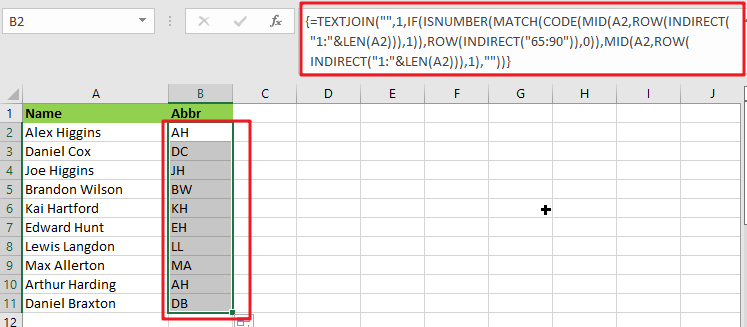So for exploring that way/method, let’s dive into the article.

## General Formula:

The array formula to abbreviate different names and words in few seconds is mentionedas follows:

`{=TEXTJOIN("",1,IF(ISNUMBER(MATCH(CODE(MID(A2,ROW(INDIRECT("1:"&LEN(A2))),1)),ROW(INDIRECT("65:90")),0)),MID(A2,ROW(INDIRECT("1:"&LEN(A2))),1),""))}`## Syntax Explanation:

To understand the working of the above formula, we first need to know about each syntax and how they contribute to abbreviating names and words in seconds.

• `TEXTJOIN`: The TEXTJOIN function in Microsoft Excel allows you to join two or more strings together, with each value separated by a delimiter. The TEXTJOIN function is an Excel built-in function classified as a String/Text Function.
• `IF`: The IF function is one of Excel’s most used functions, allowing you to create logical comparisons between a number and what you anticipate.
• `ISNUMBER`: When a cell contains a number, the ISNUMBER function returns TRUE; otherwise, it returns FALSE. ISNUMBER can be used to verify that a cell contains a numeric value or that the output of another function is a number.
• `MATCH`: The MATCH is a function used to find a lookup value in a row, column, or table in MS Excel. MATCH allows for approximate and accurate matching and wildcards (*?) for partial matches.
• `CODE`: The CODE function in Excel returns a numeric code for a specified character.
• INDIRECT: The INDIRECT function returns a range reference. This function may generate a reference that will not change if a row or column is added to the worksheet.

## Let’s See How This Formula Works:

To abbreviate capital letters text, use this array formula based on the TEXTJOIN function, new in Office 365 and Excel 2019. You may use this method to generate initials from names or acronyms. Because only capital letters can survive this algorithm, the original text must contain capitalized terms. If necessary, you can capitalize words using the PROPER function.

The formula in B2 in the case provided is:

`=TEXTJOIN("",1,IF(ISNUMBER(MATCH(CODE(MID(A2,ROW(INDIRECT("1:"&LEN(A2))),1)),ROW(INDIRECT("65:90")),0)),MID(A2,ROW(INDIRECT("1:"&LEN(A2))),1),""))`

The MID function is used to turn the string into an array of individual letters from the inside out:

`=MID(A2,ROW(INDIRECT("1:"&LEN(A2))),1)`

In this formula section, the operators MID, ROW, INDIRECT, and LEN transform a string into an array of letters.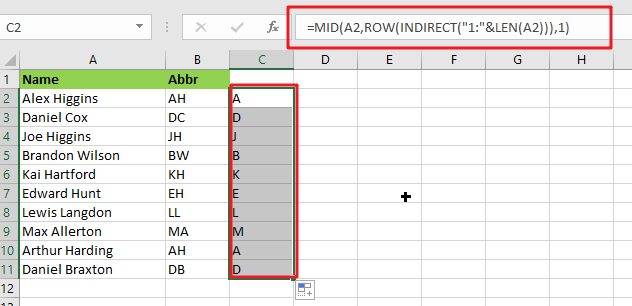MID delivers an array of all characters in the text.

`=CODE(MID(A2,ROW(INDIRECT("1:"&LEN(A2))),1))`

This above array is sent to the CODE function, which returns an array of numeric ASCII codes, one for each letter.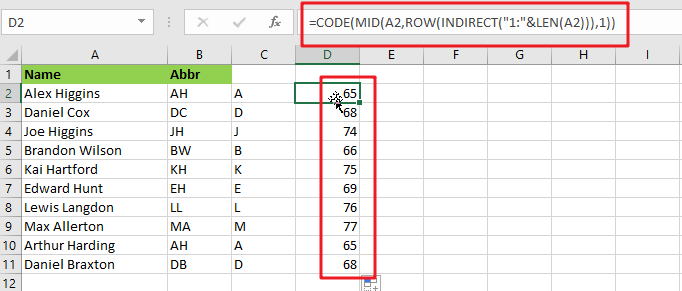`=ROW(INDIRECT("65:90")`

ROW and INDIRECT are used to generate another numeric array:

`{65;66;67;68;69;70;71;72;73;74;75;76;77;78;79;80;81;82;83;84;85;86;87;88;89;90}`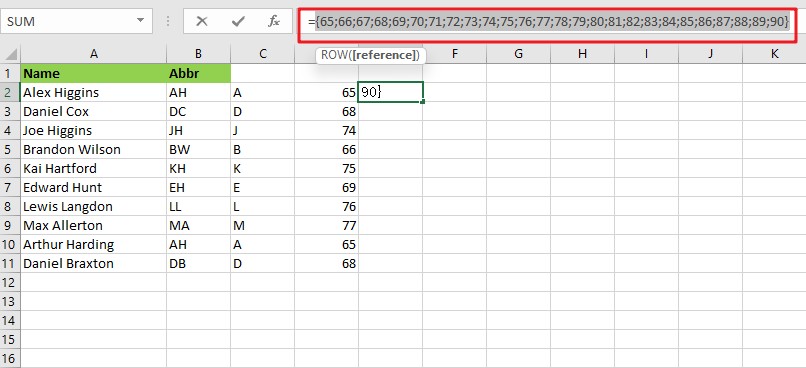Note: The digits 65 to 90 correspond to the ASCII codes for all capital letters from A to Z. This array is used as the lookup array in the MATCH function, and the original array of ASCII codes is given as the lookup value.

`{=MATCH(CODE(MID(A2,ROW(INDIRECT("1:"&LEN(A2))),1)),ROW(INDIRECT("65:90")),0)}`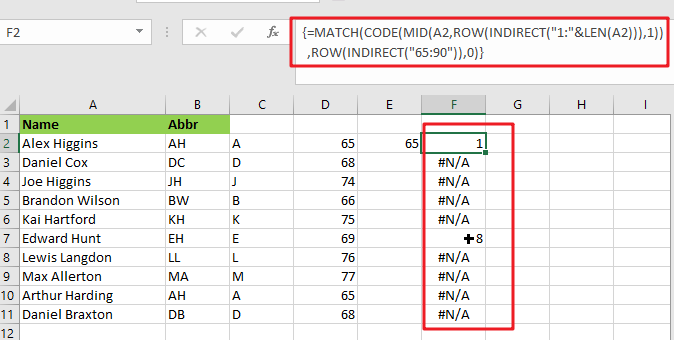The MATCH function returns either a number or the #N/A error. Because numbers represent capital letters.

`{=IF(ISNUMBER(MATCH(CODE(MID(A2,ROW(INDIRECT("1:"&LEN(A2))),1)),ROW(INDIRECT("65:90")),0)),MID(A2,ROW(INDIRECT("1:"&LEN(A2))),1),"")}`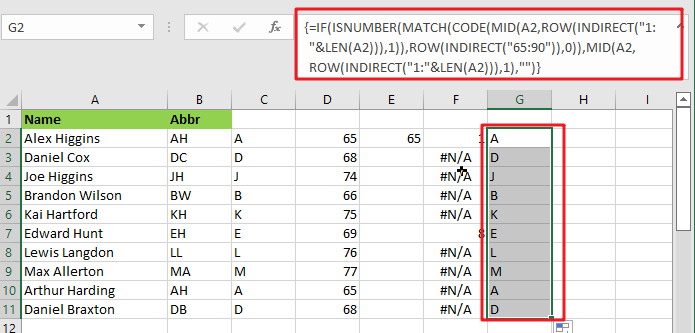The ISNUMBER function is used with the IF function to filter results. Only characters with ASCII codes between 65 and 90 will be included in the final array, reconstructed using the TEXTJOIN method to produce the final abbreviation or acronym.

## Abbreviate Names And Words In MS Excel 2016 Or Older Versions

As in Excel 2016 and earlier versions, the formula discussed above would not work, so the TRIM function is available in Excel 2016 and earlier versions, so we use it to abbreviate names and words.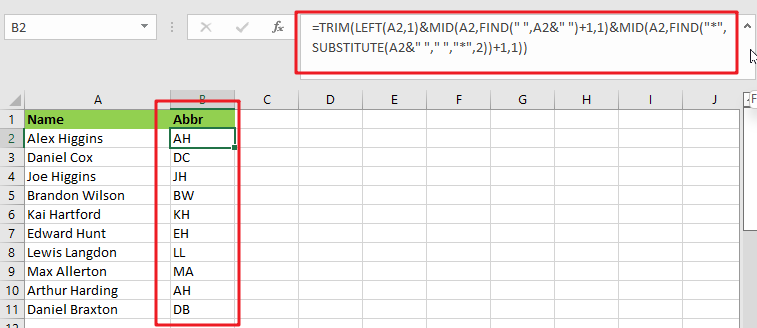### General Formula:

The formula we would use in MS Excel 2016 and earlier versions to abbreviate names and words as follows.

`=TRIM(LEFT(Text,1)&MID(Text,FIND(" ",Text&" ")+1,1)&MID(Text,FIND("*",SUBSTITUTE(Text&" ","*,"2))+1,1)`

### How Does This Formula Work?

From cell A2, if you want to extract the initials, enter this formula in cell B2.

`=TRIM(LEFT(A2,1)&MID(A2,FIND(" ",A2&" ")+1,1)&MID(A2,FIND("*",SUBSTITUTE(A2&" "," ","*",2))+1,1))`

Here the text string is the string you want to extract the first letters of each word.

When you press the Enter key, all of the first letters of each word in cell A2 are retrieved.### Explanation:

1. The TRIM function eliminates any extra spaces from the text string.
2. The LEFT(A2,1) function retrieves the first letter of the text string.
3. MID(A2,FIND(” “,A2&” “)+1,1) retrieves the initial letter of the second word separated by a space.
4. MID(A2,FIND(“*”,SUBSTITUTE(A2&” “,” “,”*,”2))+1,1) retrieves the initial letter of the third word separated by a space.

NOTE:

This formula only works when three or fewer words are in a cell. You can modify ” “ in the formula to different delimiters.

This formula extracts the initial characters in a case-insensitive manner; if you want the formula to always return in the upper case, include the UPPER function in the formula.

`=UPPER(TRIM(LEFT(A2,1)&MID(A2,FIND(" ",A2&" ")+1,1)&MID(A2,FIND("*",SUBSTITUTE(A2&" "," ","*",2))+1,1)))`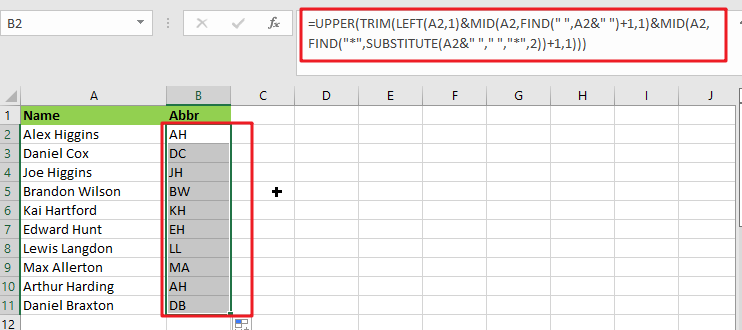### Related Functions

• Excel IF function
The Excel IF function perform a logical test to return one value if the condition is TRUE and return another value if the condition is FALSE. The IF function is a build-in function in Microsoft Excel and it is categorized as a Logical Function.The syntax of the IF function is as below:= IF (condition, [true_value], [false_value])….
• Excel TEXTJOIN function
The Excel TEXTJOIN function joins two or more text strings together and separated by a delimiter. you can select an entire range of cell references to be combined in excel 2016.The syntax of the TEXTJOIN function is as below:= TEXTJOIN  (delimiter, ignore_empty,text1,[text2])…
• Excel ROW function
The Excel ROW function returns the row number of a cell reference.The ROW function is a build-in function in Microsoft Excel and it is categorized as a Lookup and Reference Function.The syntax of the ROW function is as below:= ROW ([reference])….
• Excel MID function
The Excel MID function returns a substring from a text string at the position that you specify.The syntax of the MID function is as below:= MID (text, start_num, num_chars)…
• Excel INDIRECT function
The Excel INDIRECT function returns the cell reference based on a text string, such as: type the text string “A2” in B1 cell, it just a text string, so you can use INDIRECT function to convert text string as cell reference….
• Excel MATCH  function
The Excel MATCH function search a value in an array and returns the position of that item.The MATCH function is a build-in function in Microsoft Excel and it is categorized as a Lookup and Reference Function.The syntax of the MATCH function is as below:= MATCH  (lookup_value, lookup_array, [match_type])….
• Excel ISNUMBER function
The Excel ISNUMBER function returns TRUE if the value in a cell is a numeric value, otherwise it will return FALSE.The syntax of the ISNUMBER function is as below:= ISNUMBER (value)…
• Excel CODE function
The Excel CODE function returns the numeric ASCII value for the first character of a text string.The syntax of the CODE function is as below:= CODE  (text)…
• Excel LEN function
The Excel LEN function returns the length of a text string (the number of characters in a text string).The LEN function is a build-in function in Microsoft Excel and it is categorized as a Text Function.The syntax of the LEN function is as below:= LEN(text)…
• Excel LEFT function
The Excel LEFT function returns a substring (a specified number of the characters) from a text string, starting from the leftmost character.The LEFT function is a build-in function in Microsoft Excel and it is categorized as a Text Function.The syntax of the LEFT function is as below:= LEFT(text,[num_chars])…t)…
• Excel FIND function
The Excel FIND function returns the position of the first text string (sub string) within another text string.The syntax of the FIND function is as below:= FIND(find_text, within_text,[start_num])…
• Excel TRIM function
The Excel TRIM function removes all spaces from text string except for single spaces between words.  You can use the TRIM function to remove extra spaces between words in a string.The syntax of the TRIM function is as below:= TRIM (text)….

## How to Find and Highlight Duplicate Rows in Excel

This post will guide you how to find and highlight duplicate rows in a range of cells in Excel. How do I find duplicate rows in a range with a formula in Excel. How to find duplicate rows in two or three columns with Conditional Formatting feature in Excel.

## Find Duplicate Rows Only

If you have a list of data in two or more columns, such as: A1:C4, and you want to find duplicate rows in those columns in Excel. How to achieve it. You can use a formula based on the IF function, the SUMPRODUCT function to achieve the result of finding all duplicate rows in a range of cells. If found, returns TRUE, otherwise, returns False. Like this:

`=IF(SUMPRODUCT((\$A\$1:\$A\$4=A1)*1,(\$B\$1:\$B\$4=B1)*1,(\$C\$1:\$C\$4=C1)*1)>1,"TRUE","FALSE")`

Type this formula into Cell D1, and press Enter key in your keyboard, and then drag the AutoFill Handle over to other cells to apply this formula.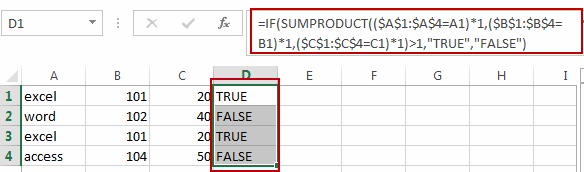## Find and Highlight Duplicate Rows

If you want to highlight duplicate rows, you need to use the conditional formatting feature to achieve the result in Excel. You just need to do the following steps:

#1 you need to create a helper column and concatenate values from all columns. Just add the below formula in Cell D1, and press Enter key, then drag the AutoFill Handle over to Cell D4 to apply the formula:

`=A1&B1&C1`

Or

`=CONCATENATE(A1,B1,C1)`

If you have a large number of columns, you can use the TEXTJOIN function to concatenate values for all columns. Like this:

`=TEXTJOIN(“,”,TRUE,A1:X1)`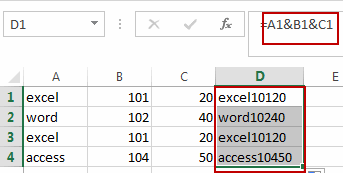#2 select the range of Cells that you want to highlight duplicate rows including the range D1:D4 in Helper column, and go to HOME tab, click Conditional Formatting command under Styles group. And click New Rule from the drop down menu list. And the New Formatting Rule dialog will open.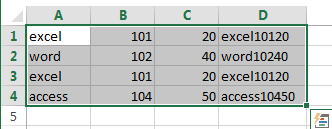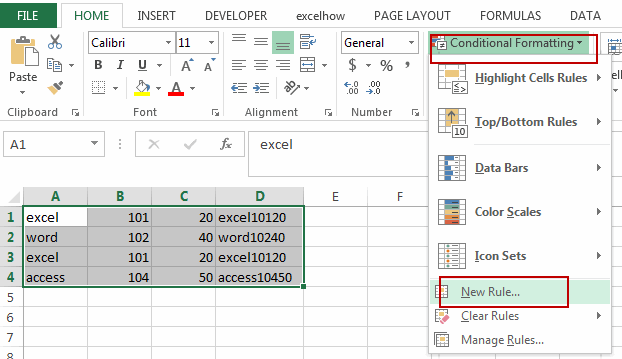#3 select Use a formula to determine which cells to format in Select a Rule Type list box, and type the following formula in text box of the Format values where this formula is true.

`=COUNTIF(\$D\$1:\$D\$4,\$D1)>1`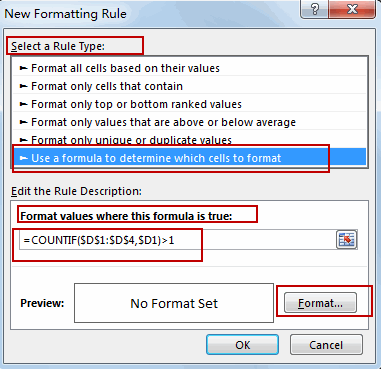D1:D4 is a range of cells in helper column.

#4 click Format command, and the Format Cells dialog will open. And switch to Fill tab, select one background color as you need, then click OK button.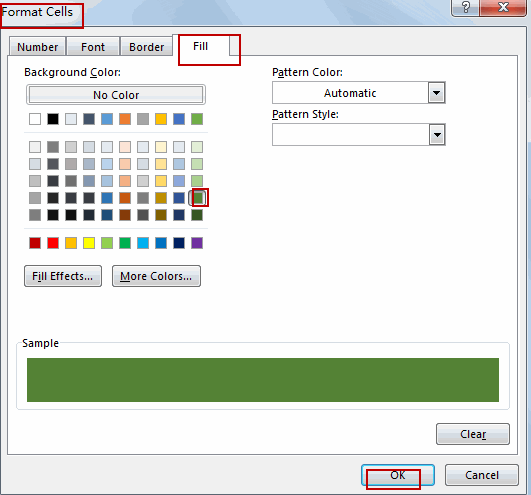#5 click OK button. All duplicate rows are highlighted successfully.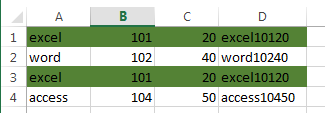Video: Find and Highlight Duplicate Rows

### Related Functions

• Excel SUMPRODUCT function
The Excel SUMPRODUCT function multiplies corresponding components in the given one or more arrays or ranges, and returns the sum of those products.The syntax of the SUMPRODUCT function is as below:= SUMPRODUCT (array1,[array2],…)…
• Excel IF function
The Excel IF function perform a logical test to return one value if the condition is TRUE and return another value if the condition is FALSE. The IF function is a build-in function in Microsoft Excel and it is categorized as a Logical Function.The syntax of the IF function is as below:= IF (condition, [true_value], [false_value])….
• Excel Concat function
The excel CONCAT function combines 2 or more strings or ranges together.This is a new function in Excel 2016 and it replaces the CONCATENATE function.The syntax of the CONCAT function is as below:=CONCAT (text1,[text2],…)…
• Excel TEXTJOIN function
The Excel TEXTJOIN function joins two or more text strings together and separated by a delimiter. you can select an entire range of cell references to be combined in excel 2016.The syntax of the TEXTJOIN function is as below:= TEXTJOIN  (delimiter, ignore_empty,text1,[text2])…
• Excel COUNTIF function
The Excel COUNTIF function will count the number of cells in a range that meet a given criteria. This function can be used to count the different kinds of cells with number, date, text values, blank, non-blanks, or containing specific characters.etc.= COUNTIF (range, criteria)…

## Reverse Text String in Excel

This post will guide you how to reverse text string in Excel. How do I reverse text string in one cell with an Excel User Defined Function. How to reverse a text string in one cell with a formula in Excel.

## Reverse Text String with User Defined Function

You can easily write down a User Defined Function to reverse a text string in one cell. Just do the following steps:

#1 open your excel workbook and then click on “Visual Basic” command under DEVELOPER Tab, or just press “ALT+F11” shortcut.#2 then the “Visual Basic Editor” window will appear.

#3 click “Insert” ->”Module” to create a new module.#4 paste the below VBA code into the code window. Then clicking “Save” button.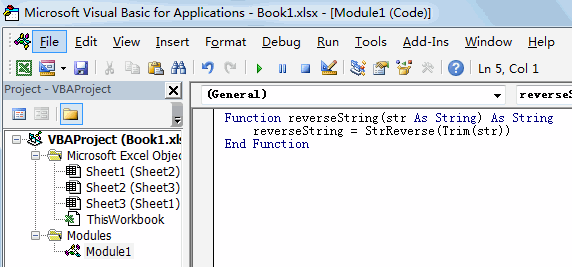Function reverseString(str As String) As String

reverseString = StrReverse(Trim(str))

End Function

#5 Type the following formula into blank cells and then press Enter key.

=reverseString(B1)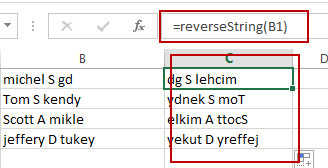## Reverse Text String with Formula

You can also use an Excel Array formula based on the TEXTJOIN function, the MID function, the ROW function, the INDIRECT function and the LEN function to reverse text string in one cell. Like this:

`=TEXTJOIN(“”,TRUE,MID(B2,LEN(B2)-ROW(INDIRECT(“1:”&LEN(\$B\$2)))+1,1))`

Type this formula into a blank cell, and then press Ctrl + Shift + Enter keys.

### Related Functions

• Excel TEXTJOIN function
The Excel TEXTJOIN function joins two or more text strings together and separated by a delimiter. you can select an entire range of cell references to be combined in excel 2016.The syntax of the TEXTJOIN function is as below:= TEXTJOIN  (delimiter, ignore_empty,text1,[text2])…
• Excel ROW function
The Excel ROW function returns the row number of a cell reference.The ROW function is a build-in function in Microsoft Excel and it is categorized as a Lookup and Reference Function.The syntax of the ROW function is as below:= ROW ([reference])….
• Excel MID function
The Excel MID function returns a substring from a text string at the position that you specify.The syntax of the MID function is as below:= MID (text, start_num, num_chars)…
• Excel INDIRECT function
The Excel INDIRECT function returns the cell reference based on a text string, such as: type the text string “A2” in B1 cell, it just a text string, so you can use INDIRECT function to convert text string as cell reference….

## Convert Column to Comma Separated List in Excel

This post will guide you how to convert column list into a comma separated list in one cell in Excel. How do I convert a column into a comma separated list with a formula in Excel. Or how to convert a column into comma delimited list in Excel.

## Convert Column to Comma Separated List in Excel

Assuming that you have a list of data in range B1:B6, and you want to convert all values in column B to comma separated list in another cell. How to achieve it.

You can create a formula based on the TEXTJOIN function to achieve the result. Just like this:

`=TEXTJOIN(“,”,TRUE,B1:B6)`

Type this formula into a blank cell and press Enter key in your keyboard.

You can also use an Excel VBA macro to quickly convert column to a comma separated list in Excel. Just do the following steps:

#1 open your excel workbook and then click on “Visual Basic” command under DEVELOPER Tab, or just press “ALT+F11” shortcut.#2 then the “Visual Basic Editor” window will appear.

#3 click “Insert” ->”Module” to create a new module.#4 paste the below VBA code into the code window. Then clicking “Save” button.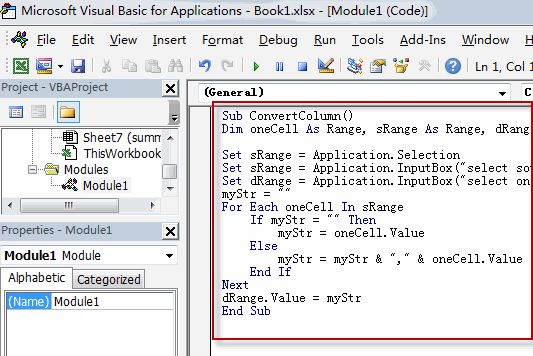```Sub ConvertColumn()
Dim oneCell As Range, sRange As Range, dRange As Range
myStr = ""

Set sRange = Application.Selection
Set sRange = Application.InputBox("select source Range:", "ConvertColumn", sRange.Address, Type:=8)
Set dRange = Application.InputBox("select one single cell as destination cell:", "ConvertColumn", Type:=8)

For Each oneCell In sRange
If myStr = "" Then
myStr = oneCell.Value
Else
myStr = myStr & "," & oneCell.Value
End If
Next
dRange.Value = myStr
End Sub```

#5 back to the current worksheet, then run the above excel macro. Click Run button.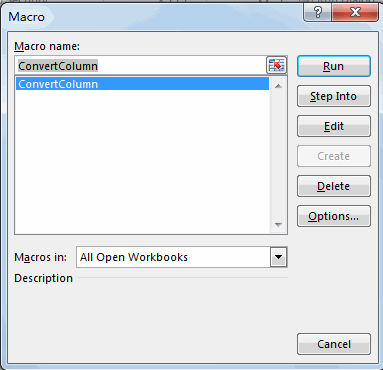#6 select the source range, such as: B1:B6.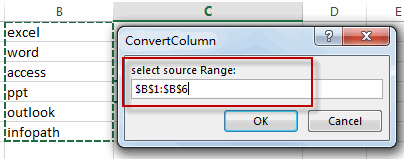#7 select one blank cell to place the list that is separated by comma. click Ok button.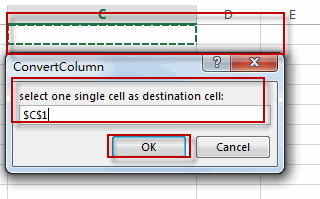#8 lets see the result.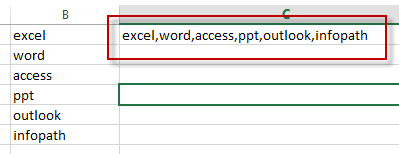## Removing Non Numeric Characters

This post will guide you how to remove non numeric characters from cells with a formula in Excel. How do I remove non-numeric characters in cells in Excel. How to remove non-numeric characters from cells with VBA Code in Excel. Or how to strip out non-numeric characters from a given cell in Excel.

## Removing Non Numeric Characters with a Formula

Assuming that you have a list of data in range B1:B5, and you want to remove all non-numeric characters from the range of cells B1:B5. How to achieve it. You can use an Excel Array formula based on the TEXTJOIN function, the IFERROR function, the ROW function and the INDIRECT function. Like this:

`=TEXTJOIN("",TRUE,IFERROR(MID(B1,ROW(INDIRECT("1:100")),1)+0,""))`

You need to Type this formula into a blank cell and then press CTRL +Shift + Enter keys to change the formula as an Array formula. and then drag the AutoFill Handle from C1 to C5.

## Removing Non Numeric Characters with User Defined Function

You can also try to write an user defined function with VBA code to remove all non numeric characters from a cell in Excel. Just do the following steps:

#1 open your excel workbook and then click on “Visual Basic” command under DEVELOPER Tab, or just press “ALT+F11” shortcut.#2 then the “Visual Basic Editor” window will appear.

#3 click “Insert” ->”Module” to create a new module.#4 paste the below VBA code into the code window. Then clicking “Save” button.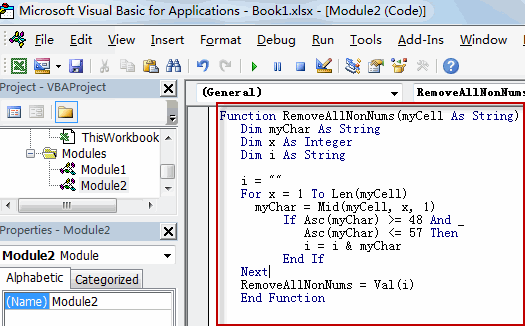```Function RemoveAllNonNums(myCell As String)
Dim myChar As String
Dim x As Integer
Dim i As String

i = ""
For x = 1 To Len(myCell)
myChar = Mid(myCell, x, 1)
If Asc(myChar) >= 48 And _
Asc(myChar) <= 57 Then
i = i & myChar
End If
Next
RemoveAllNonNums = Val(i)```

#5 back to the current worksheet, try to enter the below formula in Cell C1.

`=RemoveallNonNums(B1)`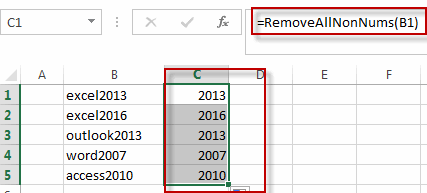You can also use the following VBA macro to achieve the same result.

```Sub RemoveAllNonNums()
Dim myR As Range
Dim myRange As Range
Set myRange = Application.Selection
Set myRange = Application.InputBox("select one range that you want to remove non-numeric characters", RemoveAllNonNums, myRange.Address, Type:=8)
For Each myR In myRange
myOut = ""
For i = 1 To Len(myR.Value)
tmp = Mid(myR.Value, i, 1)
If tmp Like "[0-9]" Then
myStr = tmp
Else
myStr = ""
End If
myOut = myOut & myStr
Next i
myR.Value = myOut
Next
End Sub```

## Removing Numeric characters only

If you only want to remove all numeric characters from a cell in Excel, and you can try to use the following array formula based on the TEXTJOIN function, the IF function, the ISERR function, the MID function, the ROW function and the INDIRECt function.

`=TEXTJOIN("",TRUE,IF(ISERR(MID(B1,ROW(INDIRECT("1:99")),1)+0),MID(B5,ROW(INDIRECT("1:99")),1),""))`

Type this formula in a blank cell and then press Ctrl + Shift + Enter shortcuts in your keyboard, and then drag the AutoFill Handle over other cells to apply this array formula.

### Related Functions

• Excel ROW function
The Excel ROW function returns the row number of a cell reference.The ROW function is a build-in function in Microsoft Excel and it is categorized as a Lookup and Reference Function.The syntax of the ROW function is as below:= ROW ([reference])….
• Excel IF function
The Excel IF function perform a logical test to return one value if the condition is TRUE and return another value if the condition is FALSE. The IF function is a build-in function in Microsoft Excel and it is categorized as a Logical Function.The syntax of the IF function is as below:= IF (condition, [true_value], [false_value])….
• Excel MID function
The Excel MID function returns a substring from a text string at the position that you specify.The syntax of the MID function is as below:= MID (text, start_num, num_chars)…
• Excel INDIRECT function
The Excel INDIRECT function returns the cell reference based on a text string, such as: type the text string “A2” in B1 cell, it just a text string, so you can use INDIRECT function to convert text string as cell reference….

## How to Combine Text from Two or More Cells into One Cell

This post explains that how to combine text from two or more cells into one cell in excel. How to concatenate the text from different cells into one cell with excel formula in excel. How to join text from two or more cells into one cell using ampersand symbol. How to combine the text using the TEXTJOIN function in excel 2016.

## Combine text using Ampersand symbol

If you want to combine text from multiple cells into one cell and you can use the Ampersand (&) symbol. For example, if you want to combine texts in the Range B1:B3 into Cell C1, Just refer to the following steps:

1# Click Cell C1 that you want to put the combined text.

2# type the below formula in the formula box of C1.

`=B1&B2&B3`

3# press Enter, you will see that the text content in B1:B3 will be joined into C1.Note:

If you want to add a space between the combined text, then you can use &” ” & instead of & in the above formula.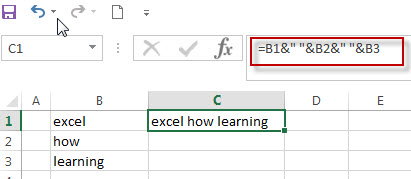And if you want to add a comma to the combined text, just type &”,”& between the combined cells in the above formula.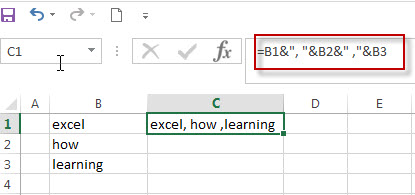If you want to add Line break between the combined cells using Ampersand operator, you should combine with the CHAR function to get the line break. Using the following formula:

`=B1 & CHAR(10) & B2 & CHAR(10) & B3`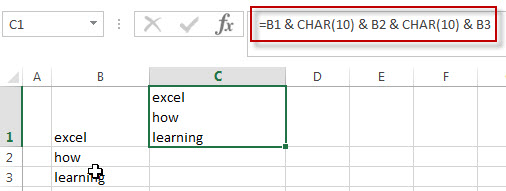## Combine text using CONCATENATE function

If you want to join the text from multiple cells into one cell, you also can use the CONCATENATE function instead of Ampersand operator. Just following the below steps to join combine the text from B1:B3 into one Cell C1.

1# Select the cell in which you want to put the combined text. (Select Cell C1)

2# type the following formula in Cell C1.

`=CONCATENATE(B1,B2,B3)`

3# press Enter to complete the formula.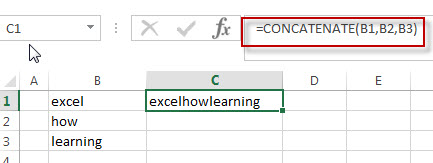Note:  If you want to add a space between the combined text strings, you can add a space character (“ “) enclosed in quotation marks. Just like the below formula:

```=CONCATENATE(B1," ",B2," ",B3)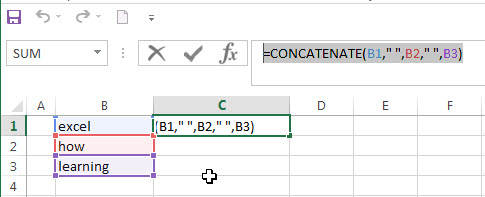```

If you want to add line break into the combined text string, you can use the CHAR function within the CONCATENATE function, just use the below formula:

`=CONCATENATE(B1,CHAR(10),B2,CHAR(10),B3)`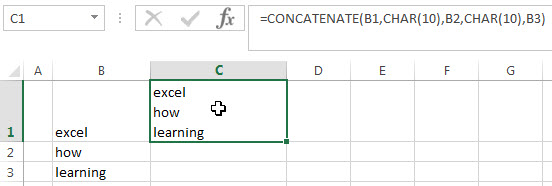## Combine text using TEXTJOIN function

If you are using the excel 2016, then you can use a new function TEXTJOIN function to combine text from multiple cells, it is only available in EXCEL 2016.  It can join the text from two or more text strings or multiple ranges into one string and also can specify a delimiter between the combined text strings. Just like the below formula:

`=TEXTJOIN(" ",TRUE,B1:B3)`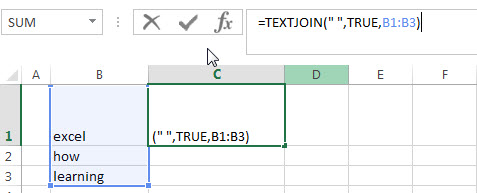### Related Formulas

• Remove Numeric Characters from a Cell
If you want to remove numeric characters from alphanumeric string, you can use the following complex array formula using a combination of the TEXTJOIN function, the MID function, the Row function, and the INDIRECT function..…
• remove non numeric characters from a cell
If you want to remove non numeric characters from a text cell in excel, you can use the array formula:{=TEXTJOIN(“”,TRUE,IFERROR(MID(B1,ROW(INDIRECT(“1:”&LEN(B1))),1)+0,””))}…

### Related Functions

• Excel Concat function
The excel CONCAT function combines 2 or more strings or ranges together.This is a new function in Excel 2016 and it replaces the CONCATENATE function.The syntax of the CONCAT function is as below:=CONCAT (text1,[text2],…)…
• Excel CHAR function
The Excel CHAR function returns the character specified by a number (ASCII Value).The CHAR function is a build-in function in Microsoft Excel and it is categorized as a Text Function. The syntax of the CHAR function is as below:=CHAR(number)….
• Excel TEXTJOIN function
The Excel TEXTJOIN function joins two or more text strings together and separated by a delimiter. you can select an entire range of cell references to be combined in excel 2016.The syntax of the TEXTJOIN function is as below:= TEXTJOIN  (delimiter, ignore_empty,text1,[text2])…

## How to Remove Numeric Characters from a Cell

This post explains that how to remove numeric characters (numbers) from a text string in one cell in excel 2016. how to remove only numeric characters but keep the non-numeric characters from a cell containing text string with an excel formula. And how to remove numbers from a cell with a user defined function in excel VBA.

## Remove numeric characters from a cell containing text

If you want to remove numeric characters from alphanumeric string, you can use the following complex array formula using a combination of the TEXTJOIN function, the MID function, the Row function, and the INDIRECT function.

`{=TEXTJOIN("",TRUE,IF(ISERR(MID(B1,ROW(INDIRECT("1":"&LEN(B1))),1)+0),MID(B1,ROW(INDIRECT("1:"&LEN(A1))),1),""))}`

Let’s see how the above array formula works:

The MID function will extract the sub strings based on the start_num and num_chars arguments. And the INDIRECT function within ROW function will returns an array list, like below:

{1,2,3…} and the length of array is the length of CELL B1.

The MID function will use the result returned by the ROW function to extract the sub string from Cell B1, as the results by the ROW function is an array, so the MID function also returns an array list. Like below format:

`{"e","x","c","e","l","2","0","1","6}`

The result returned by MID function add zero for each value in array, if the value is non-numeric value, then the ISERR function return a #VALUE error. Or return the numeric text values will be converted to number.

the IF function will check if the result returned by ISERR function, If TRUE, then return an empty string, otherwise, return that non-numeric character using the second MID function.

`{"e","x","c","e","l","","","",""}`

Last, the TEXTJOIN function will join all values and ignore the empty string.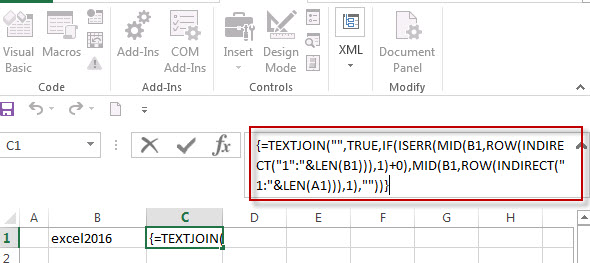## Remove numeric characters from text using a user defined function in VBA

You can create a new function to remove numeric characters from a cell that contain text string in Excel VBA. Just refer to the below steps:

1# open visual Basic Editor, then insert a module and name as : RemoveNumericCharacters.

2# click “Insert“->”Module“, then paste the following VBA code into the window: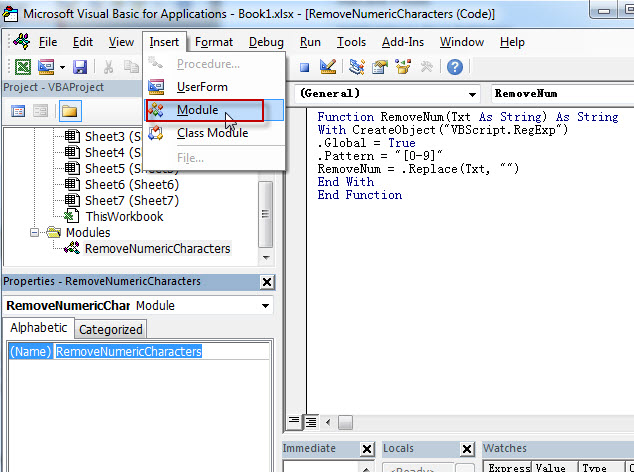```Function RemoveNum(Txt As String) As String
With CreateObject("VBScript.RegExp")
.Global = True
.Pattern = "[0-9]"
RemoveNum = .Replace(Txt, "")
End With
End Function```

3# save the user defined function.

4# enter into the below formula in Cell C1.

`=RemoveNum(B1)`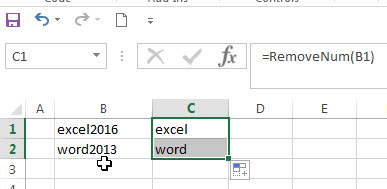### Related Formulas

• remove non numeric characters from a cell
If you want to remove non numeric characters from a text cell in excel, you can use the array formula:{=TEXTJOIN(“”,TRUE,IFERROR(MID(B1,ROW(INDIRECT(“1:”&LEN(B1))),1)+0,””))}…
• Combine Text from Two or More Cells into One Cell
If you want to combine text from multiple cells into one cell and you can use the Ampersand (&) symbol.If you are using the excel 2016, then you can use a new function TEXTJOIN function to combine text from multiple cells…

### Related Functions

• Excel TEXTJOIN function
The Excel TEXTJOIN function joins two or more text strings together and separated by a delimiter. you can select an entire range of cell references to be combined in excel 2016.The syntax of the TEXTJOIN function is as below:= TEXTJOIN  (delimiter, ignore_empty,text1,[text2])…
• Excel MID function
The Excel MID function returns a substring from a text string at the position that you specify.The syntax of the MID function is as below:= MID (text, start_num, num_chars)…
• Excel LEN function
The Excel LEN function returns the length of a text string (the number of characters in a text string). The LEN function is a build-in function in Microsoft Excel and it is categorized as a Text Function.The syntax of the LEN function is as below:= LEN(text)…
• Excel IF function
The Excel IF function perform a logical test to return one value if the condition is TRUE and return another value if the condition is FALSE.The syntax of the IF function is as below:= IF (condition, [true_value], [false_value])….
• Excel ROW function
The Excel ROW function returns the row number of a cell reference.The ROW function is a build-in function in Microsoft Excel and it is categorized as a Lookup and Reference Function.The syntax of the ROW function is as below:= ROW ([reference])….
• Excel INDIRECT  function
The Excel ROW function returns the row number of a cell reference.The ROW function is a build-in function in Microsoft Excel and it is categorized as a Lookup and Reference Function.The syntax of the ROW function is as below:= ROW ([reference])….
• Excel ISERR function
The Excel ISERR function returns TRUE if the value is any error value except #N/A.The syntax of the ISERR function is as below:= ISERR (value)…

## Excel TextJoin Function

This post will guide you how to use Excel TEXTJOIN function with syntax and examples in Microsoft excel. how to concatenate strings using the TEXTJOIN function in excel 2016. we can use CONCATENATE function to join text in differenct cells in 2013 or lower version excel.

## Description

The Excel TEXTJOIN function joins two or more text strings together and separated by a delimiter. you can select an entire range of cell references to be combined in excel 2016. and you can also specify a en empty string as delimiter to include between each texts in different cells.

The TEXTJOIN function is a build-in function in Microsoft Excel and it is categorized as a Text Function.

The TEXTJOIN function is only available in Excel 2016.

## Syntax

The syntax of the TEXTJOIN function is as below:

`= TEXTJOIN  (delimiter, ignore_empty,text1,[text2])`

Where the TEXTJOIN function arguments are:
delimiter -This is a required argument. It can be a text string or empty string to join text values with a given delimiter, it can be a space,comma,hash or other text string.
Ignore_empty – This is a required argument.  If TRUE, empty cells or string value should be ignored.
Text1/Text2– This is a required argument.  One or more strings that you want to join together.
Note: the text arguments should not exceed 252 and the length of resulting string should not exceed the cell limit of 32767.

## Excel TEXTJOIN Function Examples

The below examples will show you how to use Excel TEXTJOIN Text function to concatenate the items of one or more text strings using a specified delimiter.

#1 To join strings in B1,C1,D1 cells, just using formula:= TEXTJOIN (“,”,TRUE,B1,C1,D2).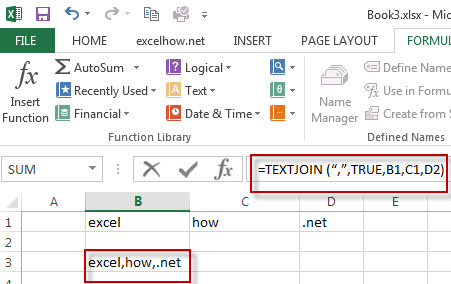#2 while you want to concatenate the strings by specifying multiple delimiter and order, you can use the following formula:

=TEXTJOIN({“@”,”.”,”.”},TRUE,A1:C1)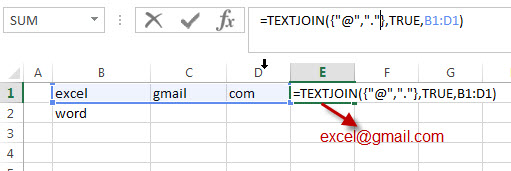#3 To join values from the multiple cell ranges with a double dash character as delimiter. using the following formula:

=TEXTJOIN(“–“,TRUE,A1:A4,B1:B4)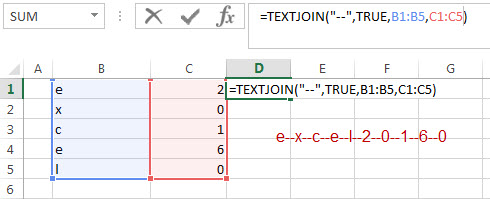#4 to join string in range cell A1:C1 by a comma character as delimiter and empty cells should not be ignored. using the following formula:

=TEXTJOIN(“,”,FALSE,A1:C1)

## More TEXTJOIN Formula Examples

• Remove Numeric Characters from a Cell
If you want to remove numeric characters from alphanumeric string, you can use the following complex array formula using a combination of the TEXTJOIN function, the MID function, the Row function, and the INDIRECT function..…
• remove non numeric characters from a cell
If you want to remove non numeric characters from a text cell in excel, you can use the array formula:{=TEXTJOIN(“”,TRUE,IFERROR(MID(B1,ROW(INDIRECT(“1:”&LEN(B1))),1)+0,””))}…
• How to combine Text from Two or More Cells into One Cell
If you want to combine text from multiple cells into one cell and you can use the Ampersand (&) symbol.If you are using the excel 2016, then you can use a new function TEXTJOIN function to combine text from multiple cells…

### Related Functions

• Excel TEXTJOIN function
The Excel TEXTJOIN function joins two or more text strings together and separated by a delimiter. you can select an entire range of cell references to be combined in excel 2016.The syntax of the TEXTJOIN function is as below:= TEXTJOIN  (delimiter, ignore_empty,text1,[text2])…
• Excel MID function
The Excel MID function returns a substring from a text string at the position that you specify.The syntax of the MID function is as below:= MID (text, start_num, num_chars)…
• Excel LEN function
The Excel LEN function returns the length of a text string (the number of characters in a text string).The LEN function is a build-in function in Microsoft Excel and it is categorized as a Text Function.The syntax of the LEN function is as below:= LEN(text)…
• Excel IFERROR function
The Excel IFERROR function returns an alternate value you specify if a formula results in an error, or returns the result of the formula.The syntax of the IFERROR function is as below:= IFERROR (value, value_if_error)….
• Excel ROW function
The Excel ROW function returns the row number of a cell reference.The ROW function is a build-in function in Microsoft Excel and it is categorized as a Lookup and Reference Function.The syntax of the ROW function is as below:= ROW ([reference])….
• Excel INDIRECT  function
The Excel ROW function returns the row number of a cell reference.The ROW function is a build-in function in Microsoft Excel and it is categorized as a Lookup and Reference Function.The syntax of the ROW function is as below:= ROW ([reference])….